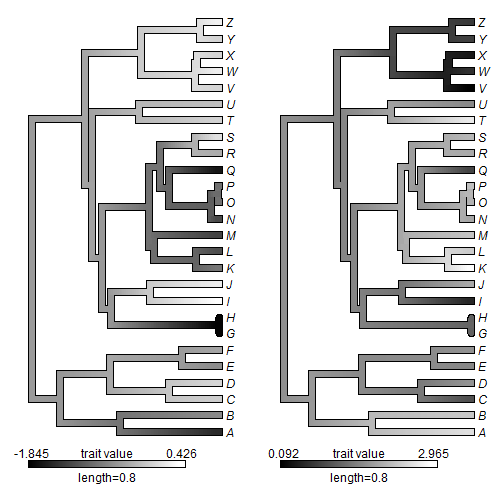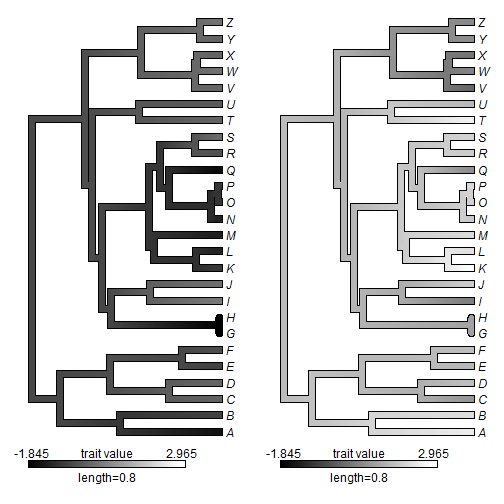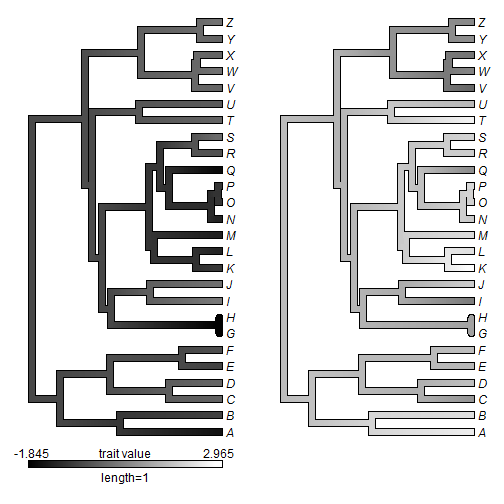## Tuesday, August 22, 2017

### Using a fixed color map for two or more plotted `"contMap"` objects

A phytools user recently submitted the following request:

“I'm writing to ask for help to make a plot for two trait with `contMap` function, and use a single legend for the two traits. The traits have the same unit, but the ranges of the values are different. When I make a plot of traits, the colors did not represent the same values (I think) and I would like to show into a unique legend the values for the two traits.

This is fairly straightforward to do using the argument `lims` as follows.

Let's imagine a phylogeny, `tree`, and two characters, `x` & `y`:

``````library(phytools)
tree
``````
``````##
## Phylogenetic tree with 26 tips and 25 internal nodes.
##
## Tip labels:
##  A, B, C, D, E, F, ...
##
## Rooted; includes branch lengths.
``````
``````x
``````
``````##           A           B           C           D           E           F
## -1.47498137 -0.61091402 -0.01033534  0.06370485 -0.59229611 -0.37826068
##           G           H           I           J           K           L
## -1.79697655 -1.84468301  0.42588201  0.26821021 -0.78436474 -1.15474354
##           M           N           O           P           Q           R
## -1.26065197 -1.21052577 -0.81250890 -0.83428720 -1.66350965 -0.30785186
##           S           T           U           V           W           X
##  0.17551957  0.03223967 -0.14868392  0.05674916  0.29106350  0.04371598
##           Y           Z
##  0.03394977  0.31722898
``````
``````y
``````
``````##          A          B          C          D          E          F
## 2.62095178 2.34889317 0.98804069 1.76638374 1.61583210 1.73510014
##          G          H          I          J          K          L
## 1.16068504 1.29077801 0.52935367 2.00890012 2.96501063 2.37973152
##          M          N          O          P          Q          R
## 2.28330800 1.95555508 2.04026749 2.33749236 0.84591649 2.00535665
##          S          T          U          V          W          X
## 2.16052968 2.74378292 1.63802572 0.09176915 0.64961048 0.35952349
##          Y          Z
## 0.82434655 0.74167574
``````

The user is right that if these two characters were plotted separately using the function `contMap` they would indeed use different color maps, determined by the range of values in each vector. For instance:

``````par(mfrow=c(1,2))
plot(setMap(contMap(tree,x,plot=F),col=c("black","white")),lwd=6,legend=0.8,
xlim=c(-0.1,1.1))
plot(setMap(contMap(tree,y,plot=F),col=c("black","white")),lwd=6,legend=0.8,
xlim=c(-0.1,1.1))
``````To force them to have the same color maps, we just need to set the argument `lims` to be a constant as follows:

``````lims<-range(c(x,y))
m1<-contMap(tree,x,plot=F,lims=lims)
m1
``````
``````## Object of class "contMap" containing:
##
## (1) A phylogenetic tree with 26 tips and 25 internal nodes.
##
## (2) A mapped continuous trait on the range (-1.844683, 2.965011).
``````
``````m2<-contMap(tree,y,plot=F,lims=lims)
m2
``````
``````## Object of class "contMap" containing:
##
## (1) A phylogenetic tree with 26 tips and 25 internal nodes.
##
## (2) A mapped continuous trait on the range (-1.844683, 2.965011).
``````

and we can plot them however we choose:

``````par(mfrow=c(1,2))
plot(setMap(m1,col=c("black","white")),lwd=6,legend=0.8,
xlim=c(-0.1,1.1))
plot(setMap(m2,col=c("black","white")),lwd=6,legend=0.8,
xlim=c(-0.1,1.1))
``````To avoid repeating the legend, we can just do this:

``````par(mfrow=c(1,2))
plot(setMap(m1,col=c("black","white")),lwd=6,legend=1,
xlim=c(-0.1,1.1))
ylim<-get("last_plot.phylo",envir=.PlotPhyloEnv)\$y.lim
plot(setMap(m2,col=c("black","white")),lwd=6,legend=F,ylim=ylim,
xlim=c(-0.1,1.1))
``````All there is to it.